## 8.4. Improved degree-day model

The purpose of modification is to use the model for a wide range of temperatures. Effective temperature (ET) is defined as follows: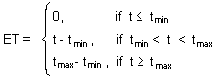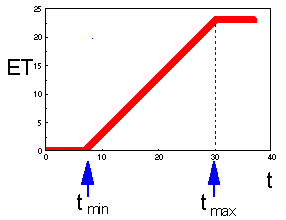This model is non-linear because the graph is not straight (see the figure above). Thus, temperature cannot be averaged! In particular, you cannot use average daily temperature. Instead, it is necessary to use actual temperature dynamics. Accumulated degree-days are equal to the area under the temperature curve restricted to the temperature interval between tmin and tmax: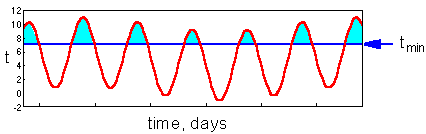Light-blue area equals to accumulated degree-days. Here the average temperature is below tmin but organisms can accumulate some degree-days because daily maximums are above tmin.

Another example: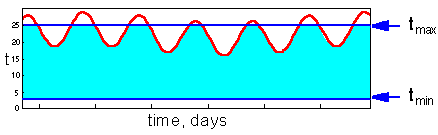Light-blue area again equals to accumulated degree-days. Daily maximum temperature exceeds tmax however this excess does not count in the accumulation of degree-days.

Non-linear models of development rate require simulation of diurnal temperature change. In most cases, only daily minimum and maximum temperature are known. There are two most frequently used methods for simulation temperature change. "Rectangular" model assumes that temperature stays at maximum for half of the day and stays at minimum for the other half: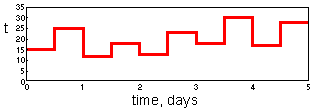This method is not very accurate but it is simple and fast.

"Sine-wave" method was developed by Allen (1976; Environ. Entomol. 5: 338-396). This model generates smooth temperature changes as in the second figure above. In the paper of Allen you will find a FORTRAN code that can be used for estimation of degree-days.

Alexei Sharov 12/5/98